# 10.2 Polar Equations of Conics

$$\newcommand{\vecs}{\overset { \rightharpoonup} {\mathbf{#1}} }$$ $$\newcommand{\vecd}{\overset{-\!-\!\rightharpoonup}{\vphantom{a}\smash {#1}}}$$$$\newcommand{\id}{\mathrm{id}}$$ $$\newcommand{\Span}{\mathrm{span}}$$ $$\newcommand{\kernel}{\mathrm{null}\,}$$ $$\newcommand{\range}{\mathrm{range}\,}$$ $$\newcommand{\RealPart}{\mathrm{Re}}$$ $$\newcommand{\ImaginaryPart}{\mathrm{Im}}$$ $$\newcommand{\Argument}{\mathrm{Arg}}$$ $$\newcommand{\norm}{\| #1 \|}$$ $$\newcommand{\inner}{\langle #1, #2 \rangle}$$ $$\newcommand{\Span}{\mathrm{span}}$$ $$\newcommand{\id}{\mathrm{id}}$$ $$\newcommand{\Span}{\mathrm{span}}$$ $$\newcommand{\kernel}{\mathrm{null}\,}$$ $$\newcommand{\range}{\mathrm{range}\,}$$ $$\newcommand{\RealPart}{\mathrm{Re}}$$ $$\newcommand{\ImaginaryPart}{\mathrm{Im}}$$ $$\newcommand{\Argument}{\mathrm{Arg}}$$ $$\newcommand{\norm}{\| #1 \|}$$ $$\newcommand{\inner}{\langle #1, #2 \rangle}$$ $$\newcommand{\Span}{\mathrm{span}}$$$$\newcommand{\AA}{\unicode[.8,0]{x212B}}$$

Polar coordinates allow you to extend your knowledge of conics in a new context. Calculators are an excellent tool for graphing polar conics. What settings do you need to know in order to properly use your calculator?

## Polar Equations of Conics

Polar equations refer to the radius $$r$$ as a function of the angle $$\theta$$. There are a few typical polar equations you should be able to recognize and graph directly from their polar form.

The following polar function is a circle of radius $$\frac{a}{2}$$ passing through the origin with a center at angle $$\beta$$.

$$r=a \cdot \cos (\theta-\beta)$$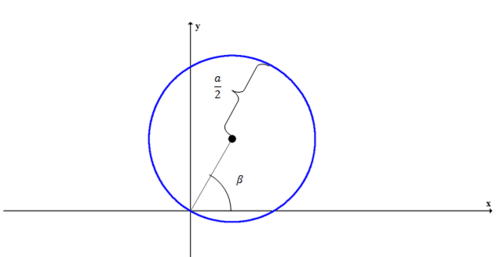There are other ways of representing a circle like this using cofunction identities and coterminal angles.

Ellipses, parabolas and hyperbolas have a common general polar equation. Just like with the circle, there are other ways of representing these relations using cofunction and coterminal angles; however, this general form is easiest to use because each parameter can be immediately interpreted in a graph. A parameter is a constant in a general equation that takes on a specific value in a specific equation.

$$r=\frac{k \cdot e}{1-e \cdot \cos (\theta-\beta)}$$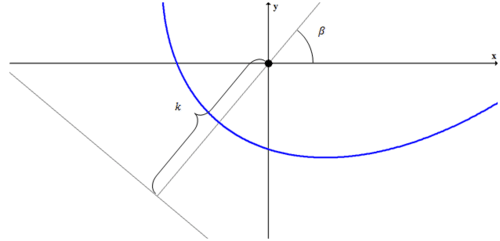One of the focus points of a conic written in this way is always at the pole (the origin). The angle $$\beta$$ indicates the angle towards the center if the conic is an ellipse, the opening direction if the conic is a parabola and the angle away from the center if the conic is a hyperbola. The eccentricity $$e$$ should tell you what conic it is. The constant $$k$$ is the distance from the focus at the pole to the nearest directrix. This directrix lies in the opposite direction indicated by $$\beta$$.

There are many opportunities for questions involving partial information with polar conics. A few relationships that are often useful for solving these questions are:

• $$e=\frac{c}{a}=\frac{\overline{P F}}{\overline{P D}} \rightarrow \overline{P F}=e \cdot \overline{P D}$$
• Ellipses: $$k=\frac{a^{2}}{c}-c$$
• Hyperbolas: $$k=c-\frac{a^{2}}{c}$$

A great way to discover new types of graphs in polar coordinates is to experiment on your own with your calculator. Try to come up with equations and graphs that look similar to the following two polar functions.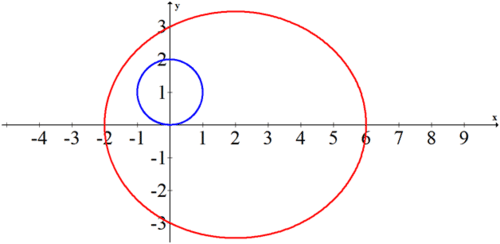The circle in blue has a center at $$90^{\circ}$$ and has a diameter of 2 . Its equation is $$r=2 \cos \left(\theta-90^{\circ}\right)$$.

The red ellipse appears to have center at (2,0) with $$a=4$$ and $$c=2$$. This means the eccentricity is $$e=\frac{1}{2} .$$ In order to write the equation in polar form you still need to find $$k$$.

$$k=\frac{a^{2}}{c}-c=\frac{4^{2}}{2}-2=8-2=6$$

Thus the equation for the ellipse is:

$$r=\frac{6 \cdot \frac{1}{2}}{1-\frac{1}{2} \cdot \cos (\theta)}$$

## Examples

##### Example 1

Earlier, you were asked about how to use your calculator to graph polar equations. Most calculators have a polar coordinate mode. On the $$\mathrm{TI}-84,$$ the mode can be switched to polar in the mode menu. This changes the graphing features. You can choose to be in radians or degrees and graphs will look the same. When you graph a circle of the form $$r=8 \cdot \cos \theta$$. you should see the following on your calculator.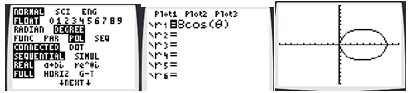When you go to the window setting you should notice that in addition to $$X_{\min }, X_{\max }$$ there are new settings called $$\theta_{\min }, \theta_{\max }$$ and $$\theta_{\text {step }}$$.

If $$\theta_{\min }$$ and $$\theta_{\max }$$ do not span an entire period, you may end up missing part of your polar graph.

The $$\theta_{\text {step }}$$ controls how accurate the graph should be. If you put $$\theta_{\text {step }}$$ at a low number like 0.1 the graph will plot extremely slowly because the calculator is doing 3600 cosine calculations. On the other hand if $$\theta_{\text {step }}=30$$ then the calculator will do fewer calculations producing a rough circle, but probably not accurate enough for your purposes.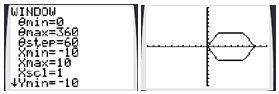##### Example 2

Identify the center, foci, vertices and equations of the directrix lines for the following conic:

$$r=\frac{20}{4-5 \cdot \cos \left(\theta-\frac{3 \pi}{4}\right)}$$

First the polar equation needs to be in graphing form. This means that the denominator needs to look like $$1-e \cdot \cos (\theta-\beta)$$.

$$r=\frac{20}{4-5 \cdot \cos \left(\theta-\frac{3 \pi}{4}\right)} \cdot \frac{\frac{1}{4}}{\frac{1}{4}}=\frac{5}{1-\frac{5}{4} \cdot \cos \left(\theta-\frac{3 \pi}{4}\right)}=\frac{4 \cdot \frac{5}{4}}{1-\frac{5}{4} \cdot \cos \left(\theta-\frac{3 \pi}{4}\right)}$$

$$e=\frac{5}{4}, \quad k=4, \quad \beta=\frac{3 \pi}{4}=135^{\circ}$$

Using this information and the relationships you were reminded of in the guidance, you can set up a system and solve for $$a$$ and $$c$$.

\begin{aligned} 4 &=c-\frac{a^{2}}{c} \\ \frac{5}{4} &=\frac{c}{a} \rightarrow \frac{4}{5}=\frac{a}{c} \rightarrow \frac{4 c}{5}=a \\ 4 &=c-\left(\frac{4 c}{5}\right)^{2} \cdot \frac{1}{c} \\ 4 &=c-\frac{16 c^{2}}{25 c} \\ 4 &=\frac{9 c}{25} \\ \frac{100}{9} &=c \\ \frac{80}{9} &=a \end{aligned}

The center is the point $$\left(\frac{100}{9}, \frac{7 \pi}{4}\right)$$ which is much more convenient to write in polar coordinates. The closest directrix is the line $$r=4 \cdot \sec \left(\theta-\frac{7 \pi}{4}\right)$$. The other directrix is the line $$r=\left(2 \cdot \frac{100}{9}-4\right) \cdot \sec \left(\theta-\frac{7 \pi}{4}\right)$$. One focus is at the pole, the other focus is the point $$\left(\frac{200}{9}, \frac{7 \pi}{4}\right)$$. The vertices are at the center plus or minus $$a$$ in the same angle:

$$\left(\frac{100}{9} \pm \frac{80}{9}, \frac{7 \pi}{4}\right)$$

Putting all this information together, the graph of the conic is: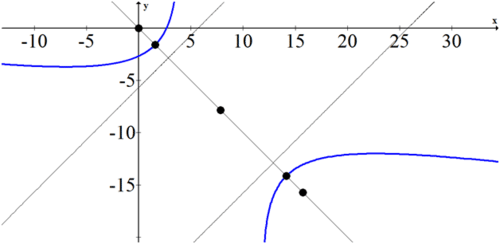##### Example 3

Convert the following conic from polar form to rectangular form.

$$r=\frac{3}{2-\cos \theta}$$

There are many ways to convert from polar form to rectangular form. You should become

comfortable with the algebra.

\begin{aligned} r &=\frac{3}{2-\cos \theta} \\ r(2-\cos \theta) &=3 \\ 2 r-r \cdot \cos \theta &=3 \\ 2 r &=3+r \cdot \cos \theta=3+y \\ 4 r^{2} &=9+6 y+y^{2} \\ 4\left(x^{2}+y^{2}\right) &=9+6 y+y^{2} \\ 4 x^{2}+4 y^{2} &=9+6 y+y^{2} \\ 4 x^{2}+3 y^{3}+6 y &=9 \\ 4 x^{2}+3\left(y^{2}+2 y+1\right) &=9+3 \\ 4 x^{2}+3(y+1)^{2} &=12 \\ \frac{x^{2}}{3}+\frac{(y+1)^{2}}{4} &=1 \end{aligned}

##### Example 4

Graph the following conic.

$$r=\frac{3}{2-\cos \left(\theta-30^{\circ}\right)}$$

Convert to the standard conic form.

\begin{aligned} r &=\frac{3}{2-\cos \left(\theta-30^{\circ}\right)} \\ r &=\frac{3}{2-\cos \left(\theta-30^{\circ}\right)} \cdot \frac{\frac{1}{2}}{\frac{1}{2}}=\frac{\frac{3}{2}}{1-\frac{1}{2} \cdot \cos \left(\theta-30^{\circ}\right)}=\frac{3 \cdot \frac{1}{2}}{1-\frac{1}{2} \cdot \cos \left(\theta-30^{\circ}\right)} \\ k &=3, \quad e=\frac{1}{2}, \quad \beta=30^{\circ} \end{aligned}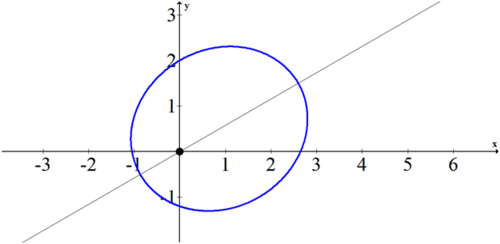##### Example 5

Translate the following conic to polar form.

$$(x-3)^{2}+(y+4)^{2}=25$$

Expand the original equation and then translate to polar coordinates:

$$(x-3)^{2}+(y+4)^{2}=25$$

$$x^{2}-6 x+9+y^{2}+8 y+16=25$$

$$r^{2}-6 x+8 y=0$$

$$r^{2}-6 r \cdot \cos \theta+8 r \cdot \sin \theta=0$$

$$r-6 \cos \theta+8 \sin \theta=0$$

$$r=6 \cos \theta-8 \sin \theta$$

##### Review

Convert the following conics from polar form to rectangular form. Then, identify the conic.

1. $$r=\frac{5}{3-\cos \theta}$$

2. $$r=\frac{4}{2-\cos \theta}$$

3. $$r=\frac{2}{2-\cos \theta}$$

4. $$r=\frac{3}{2-4 \cos \theta}$$

5. $$r=5 \cos (\theta)$$

Graph the following conics.

6. $$r=\frac{5}{4-2 \cos \left(\theta-90^{\circ}\right)}$$

7. $$r=\frac{5}{3-7 \cos \left(\theta-60^{\circ}\right)}$$

8. $$r=\frac{3}{3-3 \cos \left(\theta-30^{\circ}\right)}$$

9. $$r=\frac{1}{2-\cos \left(\theta-60^{\circ}\right)}$$

10. $$r=\frac{3}{6-3 \cos \left(\theta-45^{\circ}\right)}$$

Translate the following conics to polar form.

11. $$\frac{(x-1)^{2}}{4}+\frac{y^{2}}{3}=1$$

12. $$(x-5)^{2}+(y+12)^{2}=169$$

13. $$x^{2}+(y+1)^{2}=1$$

14. $$(x-1)^{2}+y^{2}=1$$

15. $$-3 x^{2}-4 x+y^{2}-1=0$$

This page titled 10.2 Polar Equations of Conics is shared under a CK-12 license and was authored, remixed, and/or curated by CK-12 Foundation via source content that was edited to the style and standards of the LibreTexts platform; a detailed edit history is available upon request.